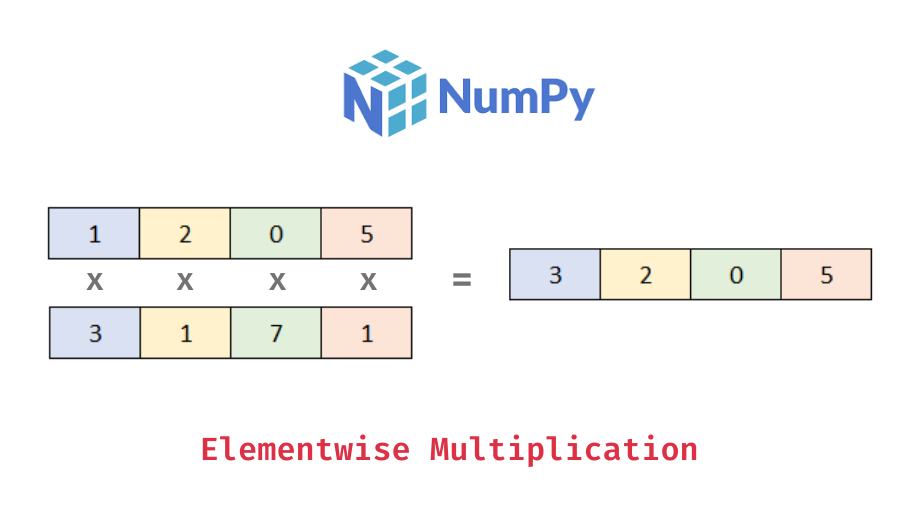# Numpy – Elementwise multiplication of two arrays

In this tutorial, we will look at how to perform elementwise multiplication of two numpy arrays with the help of some examples.

You can use the numpy `np.multiply()` function to perform the elementwise multiplication of two arrays. You can also use the `*` operator as a shorthand for `np.multiply()` on numpy arrays. The following is the syntax:

```import numpy as np
# x1 and x2 are numpy arrays of the same dimensions
# elementwise multiplication
x3 = np.multiply(x1, x2)
# elementwise multiplication using *
x3 = x1 * x2```

It returns a numpy array of the same shape with values resulting from multiplying values in each array elementwise. Note that both the arrays need to have the same dimensions.

Let’s look at some examples –

```import numpy as np

# create two 1d numpy arrays
x1 = np.array([1, 2, 0, 5])
x2 = np.array([3, 1, 7, 1])
# multiply x1 and x2 elementwise
x3 = np.multiply(x1, x2)
# display the arrays
print("x1:", x1)
print("x2:", x2)
print("x3:", x3)```

Output:

```x1: [1 2 0 5]
x2: [3 1 7 1]
x3: [3 2 0 5]```

Here, we created two one-dimensional numpy arrays of the same shape and then performed an elementwise multiplication. You can see that the resulting array, x3 has values resulting from the elementwise multiplication of values in x1 and x2.

Alternatively, you can also use the `*` operator to perform the same elementwise multiplication operation.

```# multiply x1 and x2 elementwise using *
x3 = x1 * x2
# display the arrays
print("x1:", x1)
print("x2:", x2)
print("x3:", x3)```

Output:

📚 Data Science Programs By Skill Level

Introductory

Intermediate ⭐⭐⭐

🔎 Find Data Science Programs 👨‍💻 111,889 already enrolled

Disclaimer: Data Science Parichay is reader supported. When you purchase a course through a link on this site, we may earn a small commission at no additional cost to you. Earned commissions help support this website and its team of writers.

```x1: [1 2 0 5]
x2: [3 1 7 1]
x3: [3 2 0 5]```

We get the same results as above.

You can also perform this operation on higher-dimensional arrays. For example, let’s multiply two 2d numpy arrays elementwise.

```# create two 2d numpy arrays
x1 = np.array([[1, 2],
[0, 5]])
x2 = np.array([[3, 1],
[7, 1]])
# multiply x1 and x2 elementwise
x3 = np.multiply(x1, x2)
# display the arrays
print("x1:\n", x1)
print("x2:\n", x2)
print("x3:\n", x3)```

Output:

```x1:
[[1 2]
[0 5]]
x2:
[[3 1]
[7 1]]
x3:
[[3 2]
[0 5]]```

Here, we created two 2d (2×2) numpy arrays and then performed an elementwise multiplication on their values. You can see that we get a 2d array with values resulting from an elementwise multiplication of the values in the arrays x1 and x2.

Now let’s do the same operation but using the `*` operator on arrays x1 and x2 –

```# multiply x1 and x2 elementwise using *
x3 = x1 * x2
# display the arrays
print("x1:\n", x1)
print("x2:\n", x2)
print("x3:\n", x3)```

Output:

```x1:
[[1 2]
[0 5]]
x2:
[[3 1]
[7 1]]
x3:
[[3 2]
[0 5]]```

We get the same results as above.

Let’s find out what happens if we use `np.multiply()` on two numpy arrays with different dimensions.

```# create two 1d numpy arrays
x1 = np.array([1, 2, 0, 5])
x2 = np.array([3, 1, 7, 1, 6])
# multiply x1 and x2 elementwise
x3 = np.multiply(x1, x2)```

Output:

```---------------------------------------------------------------------------
ValueError                                Traceback (most recent call last)
<ipython-input-3-3682bf62dbfa> in <module>
3 x2 = np.array([3, 1, 7, 1, 6])
4 # multiply x1 and x2 elementwise
----> 5 x3 = np.multiply(x1, x2)

ValueError: operands could not be broadcast together with shapes (4,) (5,) ```

You can see that we get an error. This happened because an elementwise operation requires the two arrays to have the same dimensions.

For more on the numpy np.multiply() function, refer to its documentation.

With this, we come to the end of this tutorial. The code examples and results presented in this tutorial have been implemented in a Jupyter Notebook with a python (version 3.8.3) kernel having numpy version 1.18.5

•